# USING QISKIT TO IMPLEMENT DEUTSCH-JOSZA’S ALGORITHM FOR CALIFORNIA HOUSING PRICE PREDICTION

Understanding quantum algorithm presupposes that one should have a clear dichotomy of what entails and what constitutes it in the simplest form. There’s no simple illustration or description one can give to quantum algorithm without getting out of bound for those with little physics and/or mathematics (linear algebra) background or experience.

While this article mainly focuses on implementation of Deutsch-Jozsa’s algorithm to predict California housing prices for some years, it will also give a thorough explanation on how Deutsch-Jozsa algorithm works. Understanding Deustch-Jozsa’s algorithm is the basis for understanding most of the quantum algorithm. The better you understand this algorithm, the easier it becomes when you face the harder ones like Grover’s, Simon’s, Shor’s algorithm etc.

I won’t be explaining how quantum gates work in this article, a better explanation is given by IBM Q Researchers and Educators. https://community.qiskit.org/education/

Enough of introduction, let’s dive into business.

Why is quantum computation such a big deal? Quantum computation exploits the inherent parallelism due to the principle of superposition of quantum states and hence has the potential to give many computational problems an exponential speedup. Most of the quantum algorithm mentioned above have been proven to some problems exponentially faster than their classical counterparts.

Deutsch-Jozsa’s algorithm is designed to solve the problem of identifying whether a binary function is constant or balanced. Its running time is 0(n) while classical method requires o(2^n).

The basic rules used in this article to implement Deutsch-Jozsa’s algorithm are as follows:

• Initialized the quantum register/circuit
• Put the registers in superposition of states
• Include unitary operators to execute the quantum algorithm
• Measure the states to get the result

There’s a quantum oracle which is mostly use to embodies step 3. Quantum oracle is similar to that of classical black box function. This oracle evaluate an unknown function

.

Given a value

the output after being executed by the oracle will be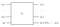Fig 1: Deutsch-Jozsa’s algorithm oracle

can either be a 1 or a 0, just like a classical bit. You might be thinking that what’s y doing in the algorithm. Well, according to quantum mechanics, a quantum oracle can only exist if it is reversible. Since quantum computers are reversible, therefore their inputs and outputs must also be reversible. Which is impossible in classical computer. So the additional input is added to create this reversible system and the output of the result is

where

denotes addition modulo 2 operator. That is, if y = 0 then the output is simply

A function

is said to be balanced if

and constant if

This might still be a little ambiguous, let’s use a table to illustrate what is being said above.

Given a function

we concluded from the function above that Deutsch-Jozsa’s algorithm will output

and make us understand if the function is balance i.e

or if it’s constant

The word balance in this sense means the output won’t be the same for every input you give the algorithm, and the word constant means it will return the same output as your input. Simple as that

Here is a table that illustrates Deutsch-Jozsa’s algorithm for a register of 3 qubits which is 8 classical bits. Input can either be one of the 8 bits below

Input = |000> |001> |010> |011> |100> |101> |110> |111>

Constant = 0 or 1 for all the inputs

If the function is balanced, the output of the eight input can either be

Balanced = |0 > |1 > |0> |1 > |0> |1 > |0> |1>

or

Balanced = |1> |0 > |1> |0 > |1> |0 > |1> |0>

For a given input, the table above give the possible output you should expect.

Let’s consider a function

that can predict whether the California housing price will increase or decrease for a given year.

x is a binary variable of 1 or 0 and years is the number of years we want to predict its value. So we want an algorithm that works this way f(x, years) = f(x). That is:

If

then California housing price will increase for that year.

If

then the California housing price will decrease for that year

If we try to do this in classical computer, we will have to try this in

queries, because we need to check every input up to

before we can make a reasonable conclusion, but a quantum computer will solve this just in 1 query.

Let’s start looking at the implementations.

Read a California housing data frame from google website, what we actually needed is the median price for each house, and for simplicity, the index of each price is the age of the house. Secondly, all necessary libraries to run the Qiskit were imported.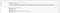Fig 2: Importing Qiskit and Python libraries

As discussed above, we want to use the principle of superposition of quantum states to create a quantum parallelism. Applying hadamard gate as denoted as h in the code below put the system into a superposition.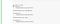Fig 3: Putting the quantum state into superposition by applying Hadamard gate

Now, let’s solve the problem. Now this code is implementing Deutsch-Jozsa’s algorithm to make a prediction. As explained earlier, if

, the housing price will increase. This is how the the quantum oracle goes. Let’s say today is 100 years as shown in the code below, if the housing price of the year we are predicting is greater than that of today, the circuit apply a NOT gate on the fourth gate. This gives us a constant value every time making sure

The second condition is that if

then apply a CNOT gate. The control of the CNOT can be on any of the three qubits, and the target on the fourth qubit.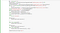Fig 4: Implementing Deutsch-Jozsa’s algorithm on a given California of data

Then give this quantum program to qasm simulator to visualize what our code is doing.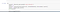Fig 5: Giving the quantum program to qasm simulator

After running the code on the simulator, it requested for 4 inputs, 3 which is binary to initialize the state of the quantum systems and the fourth input which is 21 is this case. The output shows that the Predicted value will be less than the current value. That is,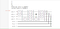Fig 6: Circuit of the quantum system

Lastly, let’s make a plot and see the output of our prediction. We input 110, we get the output of 101 in 1 shot. This shows that

is balanced, and our predicted housing price will decrease.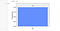Fig 7: Output plot

This article has given a simple explanation of Deutsch-Jozsa’s algorithm implementation. For further understanding of quantum computing and quantum algorithm implementation, good resources are available on QISKIT website. https://qiskit.org

Some people call me unstable force, while some say I'm quantum dot.

## More from Eniola Sobimpe

Some people call me unstable force, while some say I'm quantum dot.